Accelerate your child’s learning
Smartick is a fun way to learn math!Jul10

# Learn to Solve Measuring Problems

In this week’s post, we are going to look at measuring problems, more specifically, problems that deal with the measurements of objects.

Not only can we measure the length of a distance with just meters, centimeters, inches, or miles, but we can also use any object as the unit of measure.

For example, according to the picture below, how long is this screwdriver?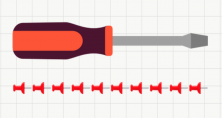In this example, the length of the screwdriver is being measured with thumbtacks. We can see that the length of the screwdriver is equivalent to 10 thumbtacks by counting how many are needed to cover its entire length. So then, we could say that the screwdriver is 10 thumbtacks long.

What are the problems that can arise from this method of measuring objects?

### Measuring Objects of Different Sizes

We can’t measure objects of different sizes because this type of measuring would be invalid. Take a look at this picture. The pencil sharpeners are different sizes, so we can’t use them for measuring.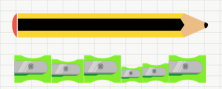### Measuring Poorly Aligned Objects

We need to be able to line up the objects that we are going to measure. In this picture here, we can see that the erasers are used for measuring, but they’re not lined up in the same position. This will give us an invalid measurement of the knife.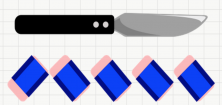### Measuring Separated Objects

It’s important to have the objects lined up in order and together. If we look at the next picture, we see that the ants are separated and we’re not able to measure the length of the pen with precision if the ants are scattered.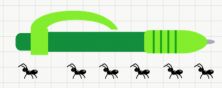Now you know the measuring problems that might occur while measuring out lengths of objects.

I’m leaving you with a measurement activity.

How long is this pencil?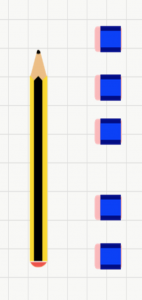(a) Exactly 5

(b) Less than 5

(c) More than 5

If you want to learn more elementary math, sign onto Smartick and try it for free!